# Overview

This vignette walks through phylogenetic semi-variograms analysis using data on Sexual Size Dimorphism (SSD) in the carnivoran superfamily Musteloidea. These trait data are paired with a time-scaled phylogenetic tree of the musteloids.

# Data import and preparation

Calculating phylogenetic variograms in ctpm requires two main pieces: i) a vector of continuous trait values; and ii) a phylogenetic tree describing the evolutionary relatedness between species within the vector of traits. To function correctly, the vector of traits needs to be of the same length and same order as the tip labels in the phylogenetic tree. This can be checked by comparing the order of the trait data against the tip.label slot of the phylo object.

Our example musteloid trait data for 48 species of extant musteloids contained within the ctpm package are already prepared. Let us first look at the trait data. Variables in this dataset include:

• Family: The family of the species.
• Binomial: The binomial of the species (Genusspecies).
• Common.Name: The common name of the species.
• Mass.M: The mean male body mass (kg).
• Mass.F: The mean female body mass (kg).
• SSD: sexual size dimorphism (male mass/female mass).
• Length.M: The mean male body length (mm).
• Length.F: The mean female body length (mm).
• Litter.Size: The mean litter size (number of offspring).
• Social.Class: The social class (Solitary, Pairs, Variable Groups, Groups).
• Diet: The diet (Carnivorous, Piscivorous, Insectivorous, Omnivorous, Frugivorous/Herbivorous).
# Load in the ctpm package
library(ctpm)

#####
#Load in the trait data and phylogenetic tree
data("moid_traits")
data("musteloids")

#Plot the trait data on a log10 - log10 scale
plot(log10(Mass.M) ~ log10(Mass.F),
data = moid_traits,
xlab = "Female mass (log10(kg))",
ylab = "Male mass (log10(kg))")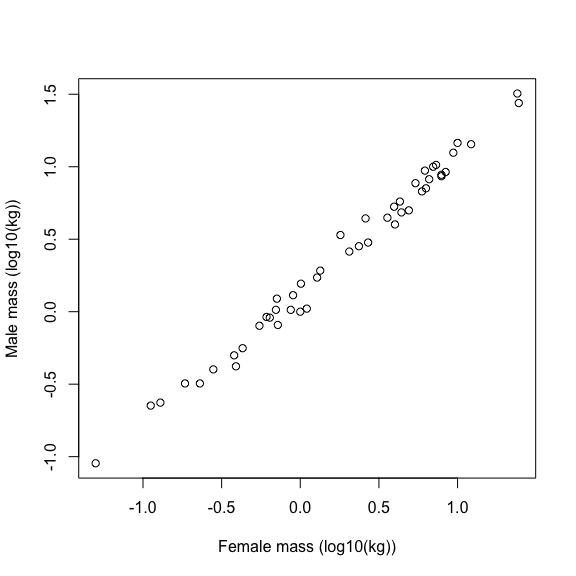From this plot we can see a correlation between male and female mass, but what can’t be seen is the underlying evolutionary process governing SSD across taxa. For this we need information on the relatedness of these species. We will therefore pair these data with a time-scaled phylogenetic tree of the musteloids, derived by:

Law, Chris J. and Slater, Graham J. and Mehta, Rita S. (2017). Lineage Diversity and Size Disparity in Musteloidea: Testing Patterns of Adaptive Radiation Using Molecular and Fossil-Based Methods. Systematic Biology, 67(1), 127-144. .

#Plot the phylogenetic tree
plot(musteloids,
cex = 0.5)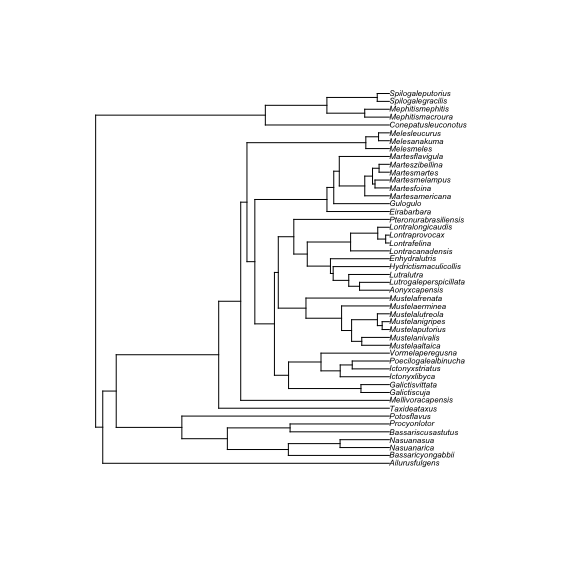From this tree we can see that the species of musteloids have diverged from one another at different times, suggesting that phylogenetic relatedness and inertia might influence the magnitude of differences in SSD we might expect to observe. From this tree alone, however, it is impossible to tell how phylogeny and SSD are correlated in time. A quick solution is to colour the tip labels based on the SSD values and check for any clustering patterns.

#Create a vector of a colour gradient of the same length as the number of species in the dataset
COLS <- viridis::viridis(nrow(moid_traits))

#Plot the phylogenetic tree with tip labels coloured by SSD values
plot(musteloids,
tip.color=COLS[order(moid_traits$SSD)], cex = 0.45)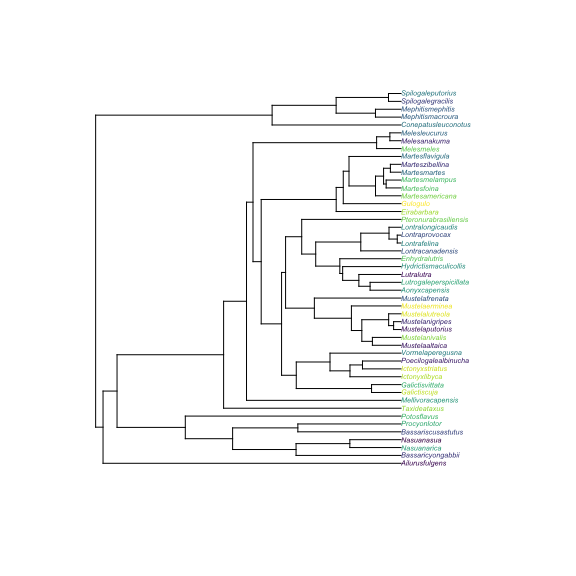There are clear visual patterns in the tree. Closely related species have similar SSD values. This suggests that SSD exhibits phylogenetic dependence and that we should try and understand the underlying evolutionary process. To do so we will use phylogenetic variograms. Variograms are an unbiased way to visualize the autocorrelation structures of evolutionary processes. # Variogram analysis We will first create a vector of the SSD values. This is not strictly necessary, but it can help shorten subsequent code. We will then check that the species trait data are correctly lined up with the tip labels in the phylogenetic tree. If these are out of order, the results will be meaningless. Finally, we will calculate the empirical variogram and plot the results. SSD <- moid_traits$SSD
names(SSD) <- moid_traits$Binomial #Check that the species are correctly lined up names(SSD) == musteloids$tip.label
##   TRUE TRUE TRUE TRUE TRUE TRUE TRUE TRUE TRUE TRUE TRUE TRUE TRUE TRUE TRUE
##  TRUE TRUE TRUE TRUE TRUE TRUE TRUE TRUE TRUE TRUE TRUE TRUE TRUE TRUE TRUE
##  TRUE TRUE TRUE TRUE TRUE TRUE TRUE TRUE TRUE TRUE TRUE TRUE TRUE TRUE TRUE
##  TRUE TRUE TRUE
#Calculate and plot the variogram
SVF <- variogram(moid_traits$SSD, musteloids, progress = F) plot(SVF)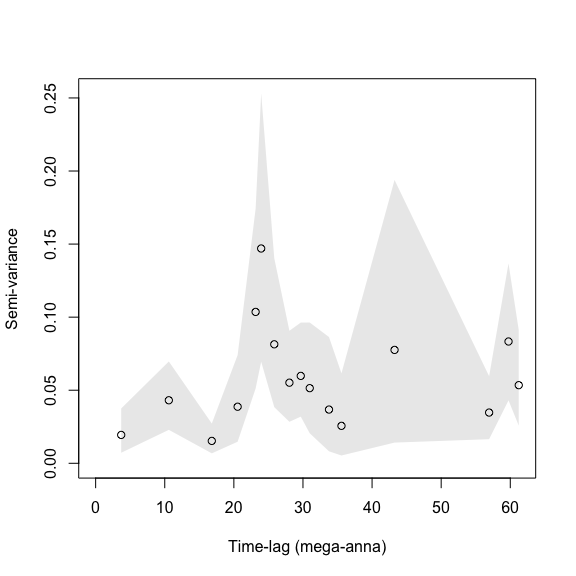While variograms can be useful on their own, we can also fit evolutionary processes to the data and compare the semi variance functions of the fitted models to the empirical variogram. The correspondence between the fitted model and the empirical variogram can then be used to determine whether a given evolutionary model might be appropriate. Eventually, the ctpm package will have its own model fitting algorithms. For the time being, however, ctpm relies on the methods implemented in the R package slouch to fit these models via the ctpm.fit wrapper function. Let’s fit IID and Brownian Motion (BM) evolutionary models to musteloid SSD and compare these against the empirical variogram. #Fit the evolutionary models IID.FIT <- ctpm.fit(SSD, musteloids, model = "IID") BM.FIT <- ctpm.fit(SSD, musteloids, model = "BM") #AIC based model selection IID.FIT$AIC
##  6.133591
BM.FIT$AIC ##  23.45008 ############## # Now plot the variograms and fitted models plot(SVF, list(IID.FIT, BM.FIT), col.CTPM = c("red", "#046C9A")) legend("topleft", fill = c("red", "#046C9A"), legend = c("IID", "BM"), horiz = T, cex = 0.8)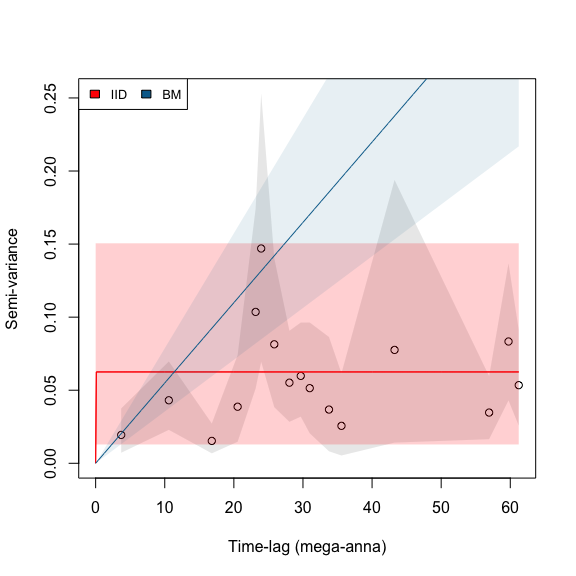AIC based model selection suggests that an IID model is a better fit than BM, but provides no information on why this model was selected over the BM processes. Comparing the fitted models against the empirical semi-variogram demonstrates the reason for the preference of the IID model over BM. The empirical semi-variogram for the variance in SSD shows clear asymptotic behaviour. The infinitely diffusive BM model was the least supported based on AICc values, and the semi-variogram clearly shows this mismatch. The IID model, in contrast, captures the asymptotic behaviour of the empirical variogram, but misses the phylogenetic autocorrelation over shorter time-scales. An Ornstein-Uhlenbeck (OU) process might be a better match here because it features phylogenetic autocorrelation over shorter time-scales but with asymptotic behaviour over long time-scales. Indeed, this is what we found to be true in Noonan et al. (2021, MEE). Unfortunately, running OU.FIT <- ctpm.fit(SSD, musteloids, model = "OU") can result in unpredictable errors due to the optimisation process that prevents the vignette from building. We encourage you to try uncommenting and running the following lines of code on your own: # #Fit the evolutionary models # IID.FIT <- ctpm.fit(SSD, musteloids, model = "IID") # BM.FIT <- ctpm.fit(SSD, musteloids, model = "BM") # OU.FIT <- ctpm.fit(SSD, musteloids, model = "OU") # # #AIC based model selection # OU.FIT$AIC
# IID.FIT$AIC # BM.FIT$AIC
#
# ##############
# # Now plot the variograms and fitted models
# plot(SVF,
#      list(IID.FIT,
#           BM.FIT,
#           OU.FIT),
#      col.CTPM = c("red",
#                   "purple",
#                   "#046C9A"))
# legend("topleft",
#        fill = c("red",
#                 "purple",
#                 "#046C9A"),
#        legend = c("IID",
#                   "BM",
#                   "OU"),
#        horiz = T,
#        cex = 0.8)